# Projection methods in linear algebra numerics

Linear algebra classes often jump straight to the definition of a projector (as a matrix) when talking about orthogonal projections in linear spaces. As often as it happens, it is not clear how that definition arises. This is what is covered in this post.

## Orthogonal projection: how to build a projector

### Case 1 – 2D projection over (1,0)

It is quite straightforward to understand that orthogonal projection over (1,0) can be practically achieved by zeroing out the second component of any 2D vector, at last if the vector is expressed with respect to the canonical basis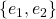. Albeit an idiotic statement, it is worth restating: the orthogonal projection of a 2D vector amounts to its first component alone.

How can this be put math-wise? Since we know that the dot product evaluates the similarity between two vectors, we can use that to extract the first component of a vector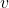. Once we have the magnitude of the first component, we only need to multiply that by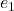itself, to know how much in the direction ofwe need to go. For example, starting from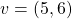, first we get the first component as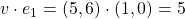; then we multiply this value by e_1 itself: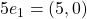. This is in fact the orthogonal projection of the original vector. Writing down the operations we did in sequence, with proper transposing, we get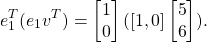One simple and yet useful fact is that when we project a vector, its norm must not increase. This should be intuitive: the projection process either takes information away from a vector (as in the case above), or rephrases what is already there. In any way, it certainly does not add any. We may rephrase our opening fact with the following proposition:

PROP 1: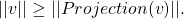This is can easily be seen through the pitagorean theorem (and in fact only holds for orthogonal projection, not oblique):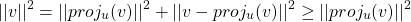### Case 2 – 2D projection over (1,1)

Attempt to apply the same technique with a random projection target, however, does not seem to work. Suppose we want to project over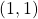. Repeating what we did above for a test vector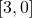, we would get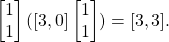This violates the previously discovered fact the norm of the projection should be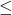than the original norm, so it must be wrong. In fact, visual inspection reveals that the correct orthogonal projection ofis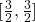.

The caveat here is that the vector onto which we project must have norm 1. This is vital every time we care about the direction of something, but not its magnitude, such as in this case. Normalizing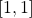yields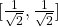. Projectingoveris obtained through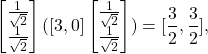which now is indeed correct!

PROP 2: The vector on which we project must be a unit vector (i.e. a norm 1 vector).

### Case3 – 3D projection on a plane

A good thing to think about is what happens when we want to project on more than one vector. For example, what happens if we project a point in 3D space onto a plane? The ideas is pretty much the same, and the technicalities amount to stacking in a matrix the vectors that span the place onto which to project.

Suppose we want to project the vector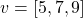onto the place spanned by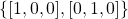. The steps are the same: we still need to know how much similaris with respect to the other two individual vectors, and then to magnify those similarities in the respective directions.The only difference with the previous cases being that vectors onto which to project are put together in matrix form, in a shape in which the operations we end up making are the same as we did for the single vector cases.

### The rise of the projector

As we have seen, the projection of a vectorover a set of orthonormal vectors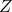is obtained as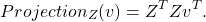And up to now, we have always done first the last product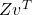, taking advantage of associativity. It should come as no surprise that we can also do it the other way around: first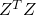and then afterwards multiply the result by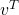. Thismakes up the projection matrix. However, the idea is much more understandable when written in this expanded form, as it shows the process which leads to the projector.

THOREM 1: The projection ofover an orthonormal basisis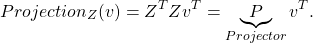So here it is: take any basis of whatever linear space, make it orthonormal, stack it in a matrix, multiply it by itself transposed, and you get a matrix whose action will be to drop any vector from any higher dimensional space onto itself. Neat.

## Projector matrix properties

• The norm of the projected vector is less than or equal to the norm of the original vector.
• A projection matrix is idempotent: once projected, further projections don’t do anything else. This, in fact, is the only requirement that defined a projector. The other fundamental property we had asked during the previous example, i.e. that the projection basis is orthonormal, is a consequence of this. This is the definition you find in textbooks: that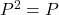. However, if the projection is orthogonal, as we have assumed up to now, then we must also have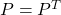.
• The eigenvalues of a projector are only 1 and 0. For an eigenvalue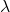,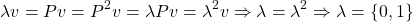• It exists a basisof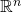such that it is possible to write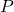as, with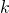being the rank of. If we further decompose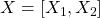, with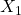being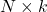and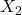being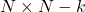, the existence of the basisshows thatreally sends points from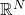into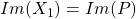and points from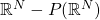into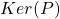. It also shows that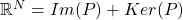.

## Model Order Reduction

Is there any application of projection matrices to applied math? Indeed.

It is often the case (or, at least, the hope) that the solution to a differential problem lies in a low-dimensional subspace of the full solution space. If some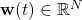is the solution to the Ordinary Differential Equation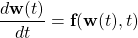then there is hope that there exists some subspace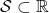, s.t.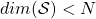in which the solution lives. If that is the case, we may rewrite it as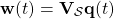for some appropriate coefficients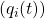, which are the components of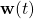over the basis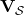.

Assuming that the base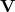itself is time-invariant, and that in general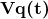will be a good but not perfect approximation of the real solution, the original differential problem can be rewritten as: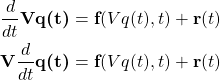where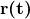is an error.